### Home > CC2 > Chapter 8 > Lesson 8.3.3 > Problem8-87

8-87.
1. Read the Math Notes box for this lesson and then find the length of the radius and diameter of each of the following circles. Homework Help ✎

2.  a.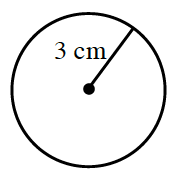b.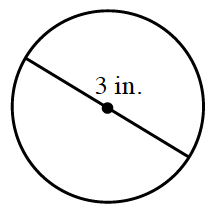c.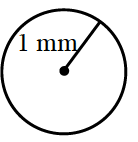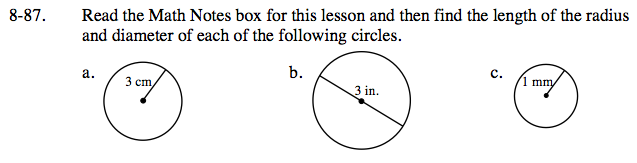Refer to the Math Notes box below in order to answer this question.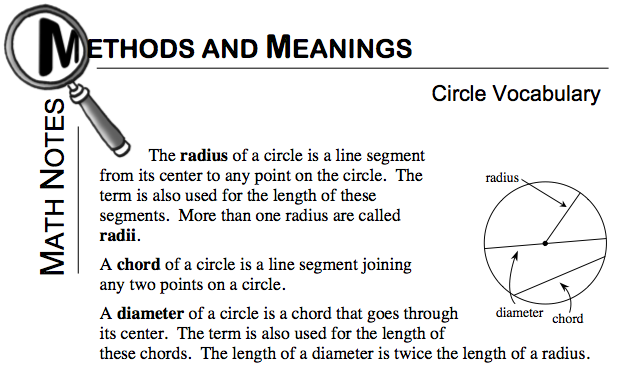Radius: 3 cm
Diameter: 6 cm

How is the information for this circle different than part (a)?

Radius: 1.5 in
Diameter: 3 in

Use the same method as part (a).